# PostgreSQL Comparison Operators

## Comparison Operators

Comparison operators, as their name, allows to comparing two values. It Compares strings or numbers for relationships such as equality.

List of Comparison Operators

Operators Description
< ( less than) Returns true when the left operand is less than the right operand.
> ( greater than) Returns true when the left operand is greater than the right operand.
<= ( less than or equal ) Returns true when the left operand is less than or equal to the right operand.
>= (greater than or equal) Returns true when the left operand is greater than or equal to the right operand.
= ( equal ) Returns true when the operands are equal but the type of the operands must be same.
<> or != ( not equal) Returns true when the operands are not equal.

The sample table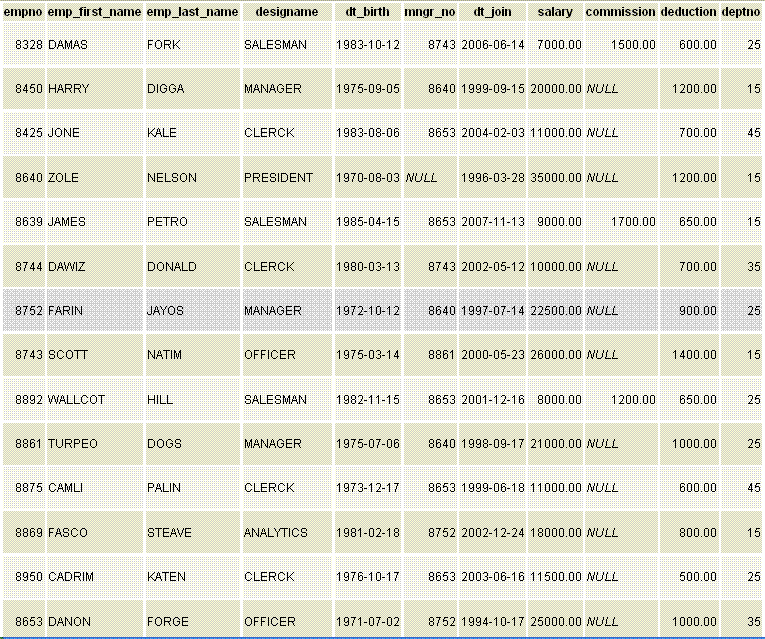PostgreSQL Less Than ( < ) operator example

If we want to display the list of employees with columns empno, emp_first_name, designame and salary from an employee who drawn the salary amount less than 10000, the following SQL can be used.

SQL

Code:

``````SELECT empno,emp_first_name,designame,salary
FROM employee
WHERE salary<10000;
```
```

Output: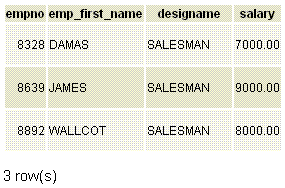PostgreSQL Greater Than ( > ) operator example

If we want to display the list of employees with columns empno, emp_first_name,designame and salary from an employee who drawn the salary amount more than 18000, the following SQL can be used.

SQL

Code:

``````SELECT empno,emp_first_name,designame,salary
FROM employee
WHERE salary>18000;
```
```

Output: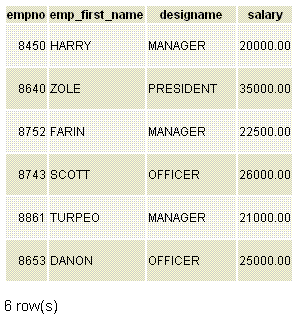PostgreSQL Greater Than or Equal( >= ) and Less Than or Equal( <= ) operator example

If we want to display the list of employees with columns empno, emp_first_name, emp_last_name,designame and dt_birth from an employee who born between the period 1975-01-01 and 1982-03-31, the following SQL can be used.

SQL

Code:

``````SELECT empno,emp_first_name,emp_last_name,designame,dt_birth
FROM employee
WHERE  dt_birth>='1975-01-01'
AND dt_birth<='1982-03-31';
```
```

Output: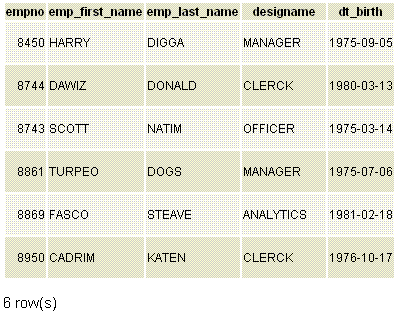PostgreSQL Equal ( = ) operator example

If we want to display the list of employees with columns empno, emp_first_name, emp_last_name and designation from employee table whose designation is 'CLERCK', the following SQL can be used.

SQL

Code:

``````SELECT empno,emp_first_name,emp_last_name,designame
FROM employee
WHERE  designame='CLERCK';
```
```

Output: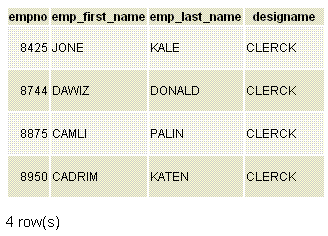PostgreSQL Not Equal (<> or != ) operator example

If we want to display the list of employees with columns empno, emp_first_name, emp_last_name and designation from employee table who does not belong to the designation 'CLERCK' and 'SALESMAN', the following SQL can be used.

SQL

Code:

``````SELECT empno,emp_first_name,emp_last_name,designame
FROM employee
WHERE  designame<>'CLERCK'
AND designame<>'SALESMAN';
```
```

Output: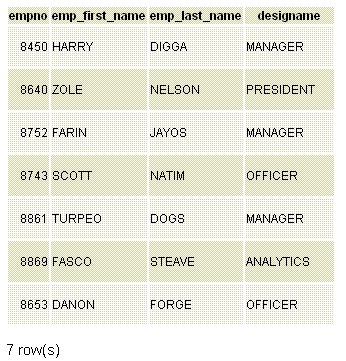Previous: Logical Operators
Next: Mathematical Operators

﻿